# Texas Go Math Grade 1 Lesson 6.5 Answer Key Practice the Strategies

Refer to our Texas Go Math Grade 1 Answer Key Pdf to score good marks in the exams. Test yourself by practicing the problems from Texas Go Math Grade 1 Lesson 6.5 Answer Key Practice the Strategies.

## Texas Go Math Grade 1 Lesson 6.5 Answer Key Practice the Strategies

Essential Question
What strategies can you use to solve addition fact problems?

Explore
Think of different addition strategies. Write or draw two ways you can solve 4 + 3.
4 + 3 = ___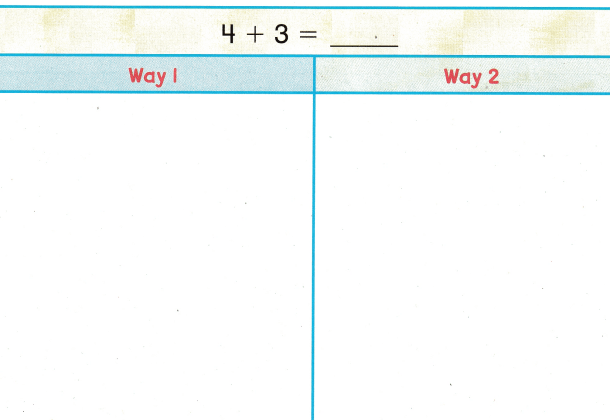For The Teacher

• Encourage children to use different strategies to show two ways they can solve 4 + 3. Have children share answers and discuss all strategies.
Explanation: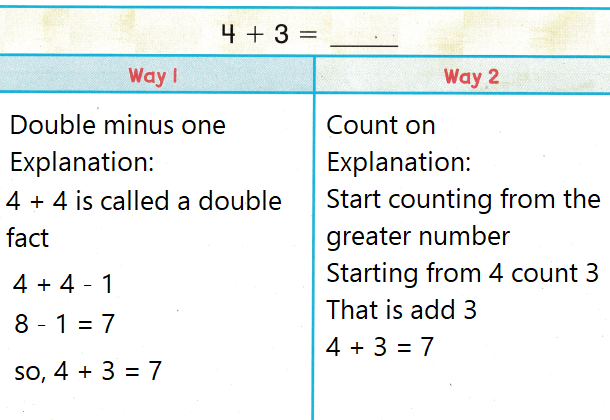Math Talk
Mathematical Processes
Explain why the sum is the same when you use different strategies.
Explanation:
The process is different but solution is same

Model and Draw

These are the ways you have learned to find sums.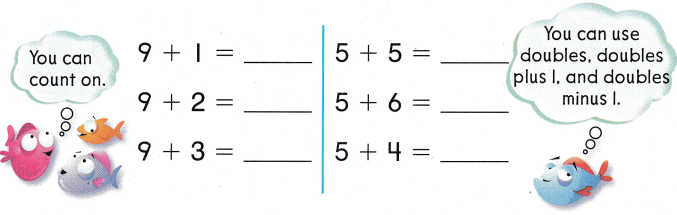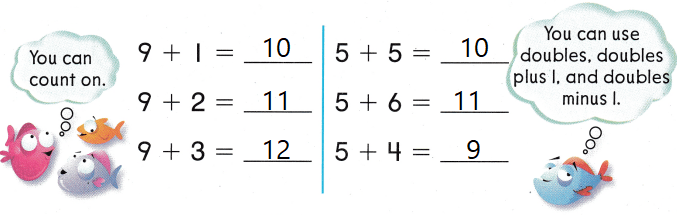Explanation:
1,2 and 3 are count on
5 + 5 = 10
is a double fact
5 + 5 + 1 = 11
is a double plus 1
5 + 5 – 1 = 9
is a double fact minus 1

Share and Show

Question 1.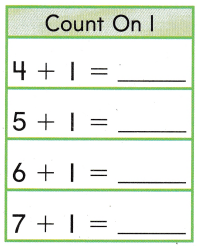Explanation:
The above box are examples of count on 1

Question 2.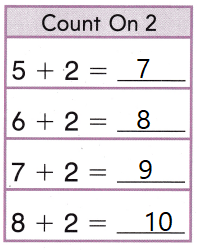Explanation:
The above box are examples of count on 2

Question 3.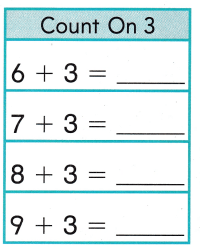Explanation:
The above box are examples of count on 3

Question 4.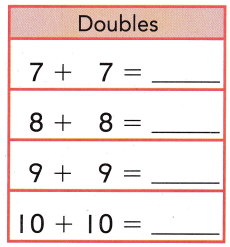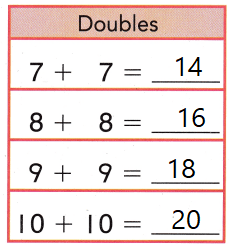Explanation:
The above box are examples of Doubles

Question 5.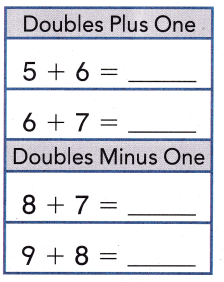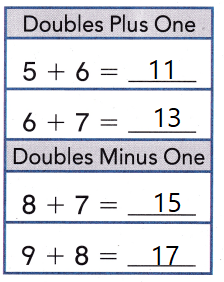Explanation:
The above box are examples of double plus 1 and double minus 1

Problem Solving

Add. Color doubles factsColor count on facts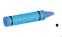Color doubles plus one or doubles minus one facts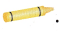Question 6.
9 + 9 = ___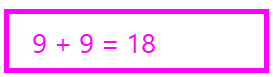Explanation:
the solution is written with colors
9 + 9
is a example of double fact
9 + 9 = 18

Question 7.
7 + 1 = ___Explanation:
the solution is written with colors
7 + 1
is a example of count on
7 + 1 = 8

Question 8.
5 + 3 = ___Explanation:
the solution is written with colors
5 + 3
is a example of count on
5 + 3 = 8

Question 9.
2 + 9 = ___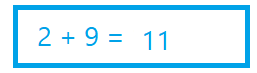Explanation:
the solution is written with colors
2 + 9
is a example of count on 2 + 9 = 11

Question 10.
7 + 3 = ___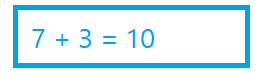Explanation:
the solution is written with colors
7 + 3
is a example of count on
7 + 3 = 10

Question 11.
7 + 8 = ___Explanation:
the solution is written with colors
7 + 8 = 15
is a example of double plus one
7 +7 + 1 = 15

Question 12.
6 + 5 = ___Explanation:
the solution is written with colors
6 + 5 = 11
is a example of double plus one
5 + 5 + 1 = 11

Question 13.
2 + 8 = ____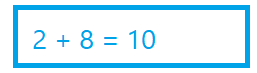Explanation:
the solution is written with colors
2 + 8 = 10
is a example of count on

Question 14.
8 + 8 = ___Explanation:
the solution is written with colors
8 +8 = 16
is a double

H.O.T. Make a counting on problem. Write the missing numbers.

Question 15.
___ birds were in a tree.
___ more birds flew there.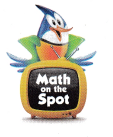How many birds are in the tree now?
Write the addition sentence to solve.
___ birds
10 birds were in a tree.
6 more birds flew there.
10 + 6 = 16
16 birds are there now.

Question 16.
H.O.T. Multi-Step There are 8 hamburger buns in 1 package. Parker bought 2 packages of hamburger buns. One package had 1 extra bun. How many hamburger buns does he have?
___ + ___ + ___ = ___ hamburger buns
8 + 8 + 1  hamburger buns
Explanation:
There are 8 hamburger buns in 1 package.
Parker bought 2 packages of hamburger buns.
8 + 8  = 16
One package had 1 extra bun.
16 + 1 = 17
17 hamburger buns that he have

Question 17.
What is the best way to solve this problem? David has 8 toy cars. He gets 2 more toy cars. How many toy cars does David have now?
(A) Count on 2.
(B) Use doubles.
(C) Use doubles minus 1.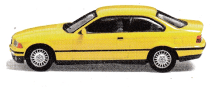Explanation:
David has 8 toy cars. He gets 2 more toy cars.
8 + 2 = 10
10 toy cars that David have now

Question 18.
Which fact is a doubles plus one fact?
(A) 8 + 1 = 9
(B) 8 + 8 = 16
(C) 8 + 9 = 17
Explanation:
8 + 9
Double plus one
8 + 8 + 1 = 17

Question 19.
Reasoning There are 5 cats at the front door. There are 4 cats at the back door. Explain how can you use the doubles fact 5 + 5 = 10 to help you find the number of cats at the doors.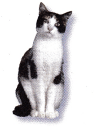Explanation:
There are 5 cats at the front door. There are 4 cats at the back door.
4 + 4 + 1 = 9
double plus one
doubles fact 5 + 5 = 10 to
5 + 5 – 1
10 – 1 = 9

Question 20.
Texas Test Prep Which is the doubles plus one fact for 6 + 6?
(A) 6 + 7 = 13
(B) 6 + 6 = 12
(C) 6 + 5 = 11
Explanation:
a number added to itself is called double fact.

Take Home Activity

• Have your child point out a doubles fact, a doubles plus one fact, a doubles minus one fact, and a fact he or she solved by counting on. Hove him or her describe how each strategy works.

### Texas Go Math Grade 1 Lesson 6.5 Homework and Practice Answer Key

Use the ways you learned to find sums.

Question 1.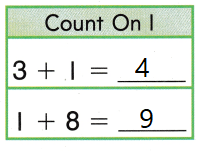Explanation:
The above box are examples of count on 1

Question 2.Explanation:
The above box are examples of count on 2

Question 3.Explanation:
The above box are examples of count on 3

Question 4.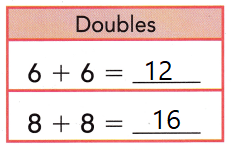Explanation:
The above box are examples of double facts

Question 5.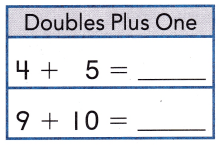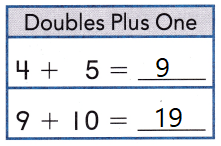Explanation:
The above box are examples of double plus 1 and double minus 1

Problem Solving

Write the addition sentence to solve.

Question 6.
Jose has 2 boxes of crayons. One box has 7 crayons. The other box has 6 crayons. How many crayons does Jose have?
___ + ___ = ___ crayons
7 + 6 = 13
Explanation:
Jose has 2 boxes of crayons. One box has 7 crayons. The other box has 6 crayons.
7 + 6 = 13
13 crayons that  Jose have

Texas Test Prep

Question 7.
What is one way to solve this problem? Casey walks 6 blocks. Then she walks another 2 blocks. How many blocks did Casey walk?
(A) Use doubles
(B) Count on 2
(C) Use doubles minus 1.
Explanation:
6 + 2
is the example of count on 2
6 + 2 = 8

Question 8.
Which doubles fact helps you solve this problem?
Ariel collects 8 leaves. Then she collects 9 more leaves.
How many leaves does Ariel have now?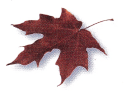(A) 9 + 9 = 18
(B) 7 + 7 = 14
(C) 10 + 10 = 20
Explanation:
doubles fact helps 9 + 9 = 18 helps to solve problem
Ariel collects 8 leaves. Then she collects 9 more leaves.
9 + 9 – 1 = 17
17 leaves that Ariel have now

Question 9.
Multi-Step Charlie had 5 toys. He bought 2 more toys. Then he bought 3 more toys. How many toys did Charlie have?
(A) 7
(B) 8
(C) 10
Explanation:
Charlie had 5 toys. He bought 2 more toys.
5 + 2 = 7
Then he bought 3 more toys.
7 + 3 = 10
10 toys that Charlie have

Question 10.
Which is the doubles minus one fact for 8 + 8?
(A) 8 + 9 = 16
(B) 8 + 6 = 14
(C) 8 + 7 = 15